# Schering Bridge (Measurement of Capacitance)

Hi friends, this post provides an information about Schering bridge which is used for measurement of capacitance. We will also see its phasor diagram, advantages and disadvantages.

The connections and phasor diagram of the Schering bridge under balance conditions are shown in figure below.

Let

• C1= capacitor whose capacitance is to be determined,

• r1 = a series resistance representing the loss in the capacitor C1

• C2 = a standard capacitor

• R3 = a non - inductive resistance

• C4 = a variable capacitor

• R4 = a variable non-inductive resistance in parallel with variable capacitor C4

Now when the Schering Bridge is balanced, then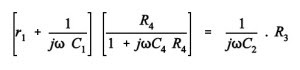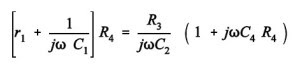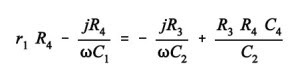By equating real and imaginary part of the equation we get,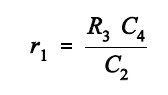Two independent balance equations are obtained if C4 and R4 are chosen as the variable elements.

The dissipation factor is given by: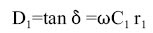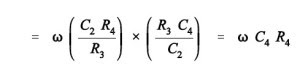Therefore values of capacitance C1 and its dissipation factor are obtained from the values of bridge elements at balance.

Permanently set up Schering bridges are sometimes arranged so that balancing is done by adjustment of R3 and C4 remaining fixed. Since R3 appears in both the balance equations and therefore there is some difficulty in obtaining balance but it has certain advantages which are explained as follows:

We know that the equation for unknown capacitance is,

In the above equation value of R4 and C2 are fixed therefore the dial resistor R3 may be calibrated to read the capacitance directly.

• 1)    The balance equation is independent of frequency.

• 2)    It is used for measuring the insulating properties of electrical cables and equipments.

You may also like: